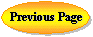Rami Arieli: "The Laser Adventure" Chapter 4 Section 4, page 14
Applet: Stability Diagram of an Optical Cavity

This applet let you change the parameters of the optical cavity of the laser:

• The radius  of curvature of each of the cavity mirrors R1 and R2.
• The cavity length L.
The computer will calculate the stability factors: g1, g2, which appear on the right side of the stability diagram.
On the stability diagram there is a red point which shows the location of the point (g1, g2).
When the red point is outside the dark region, the cavity is unstable.
On the right there is a message if the cavity is stable or not.
The structure of the cavity is shown beneath the stability diagram.
Small blue points on the cavity axis, mark the places for the center of the circles of the radius of curvature for each mirror.
The yellow region marks the active region of the cavity.

Activities:

1. Describe and explain what happened to the stability of the cavity when you increase the radius of one of the mirror from its maximum negative value (-100 in the simulation) to its maximum positive value (+100 in the simulation).

2. How is this effect shown on the stability graph?
3. For specific values of R1, and R2, What is the influence of changing the length of the cavity on the cavity stability? (Check over the entire range of cavity lengths available).